# Maharashtra Board Class 12 Physics Solutions Chapter 7 Wave Optics

Balbharti Maharashtra State Board 12th Physics Textbook Solutions Chapter 7 Wave Optics Textbook Exercise Questions and Answers.

## Maharashtra State Board 12th Physics Solutions Chapter 7 Wave Optics

1. Choose the correct option.

i) Which of the following phenomenon proves that light is a transverse wave?
(A) reflection
(B) interference
(C) diffraction
(D) polarization
(D) polarization

ii) Which property of light does not change when it travels from one medium to another?
(A) velocity
(B) wavelength
(C) amplitude
(D) frequency
(D) frequencyiii) When unpolarized light is passed through a polarizer, its intensity
(A) increases
(B) decreases
(C) remains unchanged
(D) depends on the orientation of the polarizer
(B) decreases

iv) In Young’s double slit experiment, the two coherent sources have different intensities. If the ratio of maximum intensity to the minimum intensity in the interference pattern produced is 25:1. What was the ratio of intensities of the two sources?
(A) 5:1
(B) 25:1
(C) 3:2
(D) 9:4
(D) 9:4

v) In Young’s double slit experiment, a thin uniform sheet of glass is kept in front of the two slits, parallel to the screen having the slits. The resulting interference pattern will satisfy
(A) The interference pattern will remain unchanged
(B) The fringe width will decrease
(C) The fringe width will increase
(D) The fringes will shift.
(A) The interference pattern will remain unchanged

i) What are primary and secondary sources of light?
(1) Primary sources of light: The sources that emit light on their own are called primary sources. This emission of light may be due to
(a) the high temperature of the source, e.g., the Sun, the stars, objects heated to high temperature, a flame, etc.
(b) the effect of current being passed through the source, e.g., tubelight, TV, etc.
(c) chemical or nuclear reactions taking place in the source, e.g., firecrackers, nuclear energy generators, etc.

(2) Secondary sources of light: Some sources are not self luminous, i.e., they do not emit light on their own, but reflect or scatter the light incident on them. Such sources of light are called secondary sources, e.g. the moon, the planets, objects such as humans, animals, plants, etc. These objects are visible due to reflected light.
Many of the sources that we see around are secondary sources and most of them are extended sources.

ii) What is a wavefront? How is it related to rays of light? What is the shape of the wavefront at a point far away from the source of light?
Wavefront or wave surface : The locus of all points where waves starting simultaneously from a source reach at the same instant of time and hence the particles at the points oscillate with the same phase, is called a wavefront or wave surface.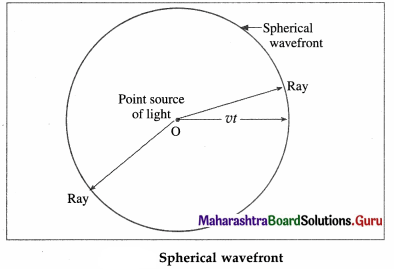Consider a point source of light O in a homogeneous isotropic medium in which the speed of light is v. The source emits light in all directions. In time t, the disturbance (light energy) from the source, covers a distance vt in all directions, i.e., it reaches out to all points which are at a distance vt from the point source. The locus of these points which are in the same phase is the surface of a sphere with the centre O and radius vt. It is a spherical wavefront.

In a given medium, a set of straight lines can be drawn which are perpendicular to the wavefront. According to Huygens, these straight lines are the rays of light. Thus, rays are always normal to the wavefront. In the case of a spherical wavefront, the rays are radial.

If a wavefront has travelled a large distance away from the source, a small portion of this wavefront appears to be plane. This part is a plane wavefront.iii) Why are multiple colours observed over a thin film of oil floating on water? Explain with the help of a diagram.
Several phenomena that we come across in our day to day life are caused by interference and diffraction of light. These are the vigorous colours of soap bubbles as well as those seen in a thin oil film on the surface of water, the bright colours of butterflies and peacocks etc. Most of these colours are not due to pigments which absorb specific colours but are due to interference of light waves that are reflected by different layers.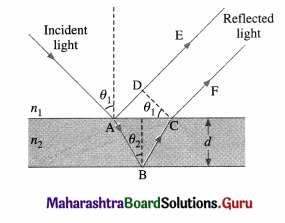Interference due to a thin film:

The brilliant colours of soap bubbles and thin films on the surface of water are due to the interference of light waves reflected from the upper and lower surfaces of the film. The two rays have a path difference which depends on the point on the film that is being viewed. This is shown in above figure.

The incident wave gets partially reflected from upper surface as shown by ray AE. The rest of the light gets refracted and travels along AB. At B it again gets partially reflected and travels along BC. At C it refracts into air and travels along CF. The parallel rays AE and CF have a phase difference due to their different path lengths in different media. As can be seen from the figure, the phase difference depends on the angle of incidence θ1, i.e., the angle of incidence at the top surface which is the angle of viewing, and also on the wavelength of the light as the refractive index of the material of the thin film depends on it. The two waves propagating along AE and CF interfere producing maxima and minima for different colours at different angles of viewing. One sees different colours when the film is viewed at different angles.

As the reflection is from the denser boundary, there is an additional phase difference of π radians (or an
additional path difference λ). This should be taken into account for mathematical analysis.

iv) In Young’s double slit experiment what will we observe on the screen when white light is incident on the slits but one slit is covered with a red filter and the other with a violet filter? Give reasons for your answer.
In Young’s double-slit experiment, when white light is incident on the slits and one of the slit is covered with a red filter, the light passing through this slit will emerge as the light having red colour. The other slit which is covered with a violet filter, will give light having violet colour as emergent light. The interference fringes will involve mixing of red and violet colours. At some points, fringes will be red if constructive interference occurs for red colour and destructive interference occurs for violet colour. At some points, fringes will be violet if constructive interference occurs for violet colour and destructive interference occurs for red colour. The central fringe will be bright with mixing of red and violet colours.

v) Explain what is optical path length. How is it different from actual path length?
Consider, a light wave of angular frequency ω and wave vector k travelling through vacuum along the x-direction. The phase of this wave is (kx-ωt). The speed of light in vacuum is c and that in medium is v.
k = $$\frac{2 \pi}{\lambda}$$ = $$\frac{2 \pi v}{v \lambda}$$ = $$\frac{\omega}{v}$$ as ω = 2πv and v = vλ, where v is the frequency of light.

If the wave travels a distance ∆x, its phase changes by ∆φ = k∆x = ω∆x/v.
Similarly, if the wave is travelling in vacuum, k = ω/c and ∆φ = ω∆x/c
Now, consider a wave travelling a distance ∆x in the medium, the phase difference generated is,
∆φ’ = k’∆x = ωn∆x/c = ω∆x’/c … (1)
where ∆x’ = n∆x … (2)
The distance n∆x is called the optical path length of the light in the medium; it is the distance the light would have travelled in the same time t in vacuum (with the speed c).

The optical path length in a medium is the corresonding path in vacuum that the light travels in the same time as it takes in the given medium.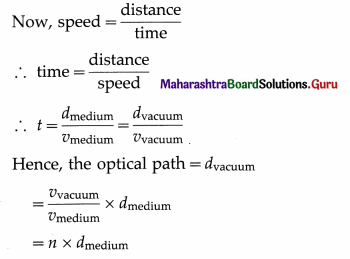Thus, a distance d travelled in a medium of refractive index n introduces a path difference = nd – d = d(n – 1) over a ray travelling equal distance through vacuum.

Question 3.
Derive the laws of reflection of light using Huygens’ principle.
Consider a plane wavefront AB of monochromatic light propagating in the direction A’A incident obliquely at an angle i on a plane refracting surface MN. This plane refracting surface MN separates two uniform and optically transparent mediums.
Let v1 and v2 be the speeds of light in medium 1 (say, a rarer medium) and medium 2 (a denser medium) respectively.When the wavefront reaches MN at point A at t = 0, A becomes a secondary source and emits secondary waves in the second medium, while ray B’B reaches the surface MN at C at time t = T. Thus, BC = v1T. During the time T, the secondary wavelet originating at A covers a distance AE in the denser medium with radius v2T.

As all the points on CE are in the same phase of wave motion, CE represents the refracted wavefront in the denser medium. CE is the tangent to the secondary wavelet starting from A. It is also a common tangent to all the secondary wavelets emitted by points between A and C. PP’ is the normal to the boundary at A.
∠A’AP = ∠BAC = the angle of incidence (i) and ∠P’AE = ∠ACE = the angle of refraction (r).
From ∆ABC and ∆AEC,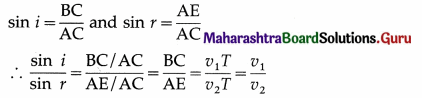By definition, the refractive index of medium 2 with respect to medium 1,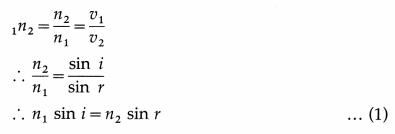Here, n1 and n2 are the absolute refractive indices of medium 1 and medium 2 respectively. Eq. (1) is Snell’s law of refraction. Also, it can be seen from the figure, that the incident ray and the refracted ray lie on the opposite sides of the normal and all three of them lie in the same plane.

Thus, the laws of refraction of light can be deduced by Huygens’ construction of a plane wavefront.
If v1 > v2, i.e. n1 < n2, then r < i (bending of the refracted ray towards the normal).
[Notes :
(1) Quite often, the terms vacuum and free space are used in the same sense. Absolute vacuum or a perfect vacuum-a region of space devoid of material, particles – does not exist. The term vacuum is also used to mean a region of space occupied by a gas at very low pressure. Free space means a region of space devoid of matter and fields. Its refractive index is 1 (by definition). Its temperature is 0 K. ε0 and μ0 are defined for free space. The refractive index of a medium with respect to air is very close to the absolute refractive index of the medium as the speed of light in air is very close to that in free space.
(2) There is no lateral inversion in refraction.
(3) There is no bending of light when the angle of incidence is zero (normal incidence), r = 0 for i = 0.]Question 4.
Derive the laws of refraction of light using Huygens’ principle.
Consider a plane wavefront AB of monochromatic light propagating in the direction A’A incident obliquely at an angle i on a plane refracting surface MN. This plane refracting surface MN separates two uniform and optically transparent mediums.
Let v1 and v2 be the speeds of light in medium 1 (say, a rarer medium) and medium 2 (a denser
medium) respectively.When the wavefront reaches MN at point A at t = 0, A becomes a secondary source and emits secondary waves in the second medium, while ray B’B reaches the surface MN at C at time t = T. Thus, BC = v1T. During the time T, the secondary wavelet originating at A covers a distance AE in the denser medium with radius v2T.

As all the points on CE are in the same phase of wave motion, CE represents the refracted wavefront in the denser medium. CE is the tangent to the secondary wavelet starting from A. It is also a common tangent to all the secondary wavelets emitted by points between A and C. PP’ is the normal to the boundary at A.
∠A’AP = ∠BAC = the angle of incidence (i) and ∠P’AE = ∠ACE = the angle of refraction (r).
From ∆ABC and ∆AEC,By definition, the refractive index of medium 2 with respect to medium 1,Here, n1 and n2 are the absolute refractive indices of medium 1 and medium 2 respectively. Eq. (1) is Snell’s law of refraction. Also, it can be seen from the figure, that the incident ray and the refracted ray lie on the opposite sides of the normal and all three of them lie in the same plane.

Thus, the laws of refraction of light can be deduced by Huygens’ construction of a plane wavefront.
If v1 > v2, i.e. n1 < n2, then r < i (bending of the refracted ray towards the normal).
[Notes :

1. Quite often, the terms vacuum and free space are used in the same sense. Absolute vacuum or a perfect vacuum-a region of space devoid of material, particles – does not exist. The term vacuum is also used to mean a region of space occupied by a gas at very low pressure. Free space means a region of space devoid of matter and fields. Its refractive index is 1 (by definition). Its temperature is 0 K. ε0 and μ0 are defined for free space. The refractive index of a medium with respect to air is very close to the absolute refractive index of the medium as the speed of light in air is very close to that in free space.
2. There is no lateral inversion in refraction.
3. There is no bending of light when the angle of incidence is zero (normal incidence), r = 0 for i = 0.]

Question 5.
Explain what is meant by polarization and derive Malus’ law.
According to the electromagnetic theory of light, a light wave consists of electric and magnetic fields vibrating at right angles to each other and to the direction of propagation of the wave. If the vibrations of $$\vec{E}$$ in a light wave are in all directions perpendicular to the direction of propagation of light, the wave is said to be unpolarized.

If the vibrations of the electric field $$\vec{E}$$ in a light wave are confined to a single plane containing the direction of propagation of the wave so that its electric field is restricted along one particular direction at right angles to the direction of propagation of the wave, the wave is said to be plane-polarized or linearly polarized.

This phenomenon of restricting the vibrations of light, i.e., of the electric field vector in a particular direction, which is perpendicular to the direction of the propagation of the wave is called polarization of light.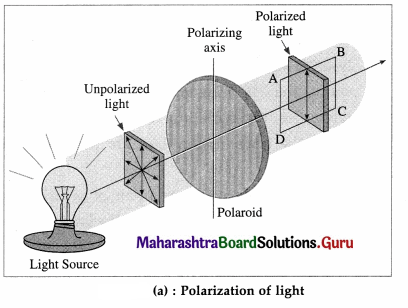Consider an unpolarized light wave travelling along the x-direction. Let c, v and λ be the speed, frequency and wavelength, respectively, of the wave. The magnitude of its electric field ($$\vec{E}$$) is,
E = E0 sin (kx – ωt), where E0 = Emax = amplitude of the wave, ω = 2πv = angular frequency of the wave and k = $$\frac{2 \pi}{\lambda}$$ = magnitude of the wave vector or propagation vector.

The intensity of the wave is proportional to $$\left|E_{0}\right|^{2}$$. The direction of the electric field can be anywhere in the y-z plane. This wave is passed through two identical polarizers as shown in below figure.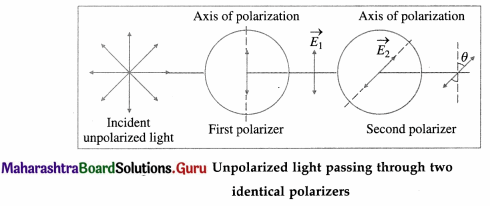When a wave with its electric field inclined at an angle φ to the axis of the first polarizer is passed through the polarizer, the component E0 cos φ will pass through it. The other component E0 sin φ which is perpendicular to it will be blocked.

Now, after passing through this polarizer, the intensity of this wave will be proportional to the square of its amplitude, i.e., proportional to |E0 cos φ|2.

The intensity of the plane-polarized wave emerging from the first polarizer can be obtained by averaging | E0 cos φ|2 over all values of φ between 0 and 180°. The intensity of the wave will be
proportional to $$\frac{1}{2}$$|E0|2 as the average value of cos2 φ over this range is $$\frac{1}{2}$$. Thus the intensity of an unpolarized wave reduces by half after passing through a polarizer.

When the plane-polarized wave emerges from the first polarizer, let us assume that its electric field ($$\overrightarrow{E_{1}}$$) is along the y-direction. Thus, this electric field is,
$$\overrightarrow{E_{1}}$$ = $$\hat{\mathrm{j}}$$ E10 sin (kx – ωt) …. (1)
where, E10 is the amplitude of this polarized wave. The intensity of the polarized wave,
I1 ∝ |E10|2 …(2)

Now this wave passes through the second polarizer whose polarization axis (transmission axis) makes an angle θ with the y-direction. This allows only the component E10 cos θ to pass through it. Thus, the amplitude of the wave which passes through the second polarizer is E20 = E10 cos θ and its intensity,
I2 ∝ | E20|2
∴ I2 ∝ | E10|2 cos2θ
∴ I2 = I1 cos2θ … (3)

Thus, when plane-polarized light of intensity I1 is incident on the second identical polarizer, the intensity of light transmitted by the second polarizer varies as cos2θ, i.e., I2 = I1 cos2θ, where θ is the angle between the transmission axes of the two polarizers. This is known as Malus’ law

[Note : Etienne Louis Malus (1775-1812), French military engineer and physicist, discovered in 1809 that light can be polarized by reflection. He was the first to use the word polarization, but his arguments were based on Newton’s corpuscular theory.]Question 6.
What is Brewster’s law? Derive the formula for Brewster angle.
Brewster’s law : The tangent of the polarizing angle is equal to the refractive index of the reflecting medium with respect to the surrounding (1n2). If θB is the polarizing angle,
tan θB = 1n2 = $$\frac{n_{2}}{n_{1}}$$
Here n1 is the absolute refractive index of the surrounding and n2 is that of the reflecting medium.
The angle θB is called the Brewster angle.

Consider a ray of unpolarized monochromatic light incident at an angle θB on a boundary between two transparent media as shown in below figure. Medium 1 is a rarer medium with refractive index n1 and medium 2 is a denser medium with refractive index n2. Part of incident light gets refracted and the rest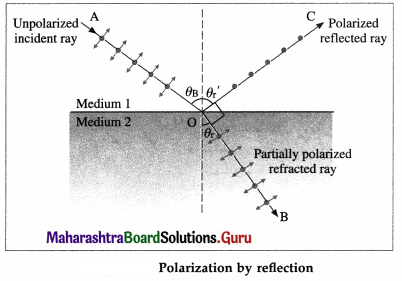gets reflected. The degree of polarization of the reflected ray varies with the angle of incidence.
The electric field of the incident wave is in the plane perpendicular to the direction of propagation of incident light. This electric field can be resolved into a component parallel to the plane of the paper, shown by double arrows, and a component perpendicular to the plane of the paper shown by dots, both having equal magnitude. Generally, the reflected and refracted rays are partially polarized, i.e., the two components do not have equal magnitude.

In 1812, Sir David Brewster discovered that for a particular angle of incidence. θB, the reflected wave is completely plane-polarized with its electric field perpendicular to the plane of the paper while the refracted wave is partially polarized. This particular angle of incidence (θB) is called the Brewster angle.

For this angle of incidence, the refracted and reflected rays are perpendicular to each other.
For angle of refraction θr,
θB + θr = 90° …… (1)
From Snell’s law of refraction,
∴ n1 sin θB = n2 sin θr … (2)
From Eqs. (1) and (2), we have,
n1 sin θB = n2 sin (90° – θB) = n2 cos θB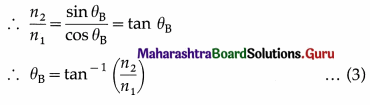This is called Brewster’s law.

Question 7.
Describe Young’s double slit interference experiment and derive conditions for occurrence of dark and bright fringes on the screen. Define fringe width and derive a formula for it.
Description of Young’s double-slit interference experiment:

1. A plane wavefront is obtained by placing a linear source S of monochromatic light at the focus of a convex lens. It is then made to pass through an opaque screen AB having two narrow and similar slits S1 and S2. S1 and S2 are equidistant from S so that the wavefronts starting simultaneously from S and reaching S1 and S2 at the same time are in phase. A screen PQ is placed at some distance from screen AB as shown in below figure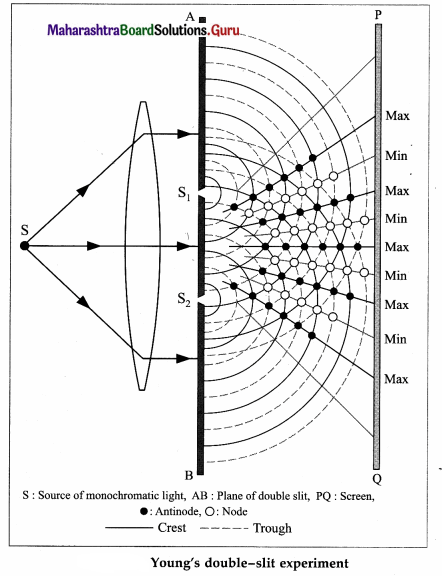2. S1 and S2 act as secondary sources. The crests/-troughs of the secondary wavelets superpose and interfere constructively along straight lines joining the black dots shown in above figure. The point where these lines meet the screen have high intensity and are bright.
3. Similarly, there are points shown with red dots where the crest of one wave coincides with the trough of the other. The corresponding points on the screen are dark due to destructive interference.
4. These dark and bright regions are called fringes or bands and the whole pattern is called interference pattern.

Conditions for occurence of dark and bright fringes on the screen :

Consider Young’s double-slit experimental set up. Two narrow coherent light sources are obtained by wavefront splitting as monochromatic light of wavelength λ emerges out of two narrow and closely spaced, parallel slits S1 and S2 of equal widths. The separation S1S2 = d is very small. The interference pattern is observed on a screen placed parallel to the plane of and at considerable distance D (D » d) from the slits. OO’ is the perpendicular bisector of segment S1S2.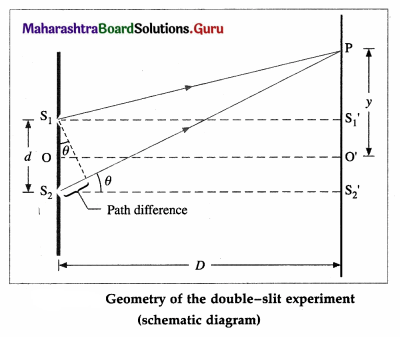Consider, a point P on the screen at a distance y from O’ (y « D). The two light waves from S1 and S2 reach P along paths S1P and S2P, respectively. If the path difference (∆l) between S1P and S2P is an integral multiple of λ, the two waves arriving there will interfere constructively producing a bright fringe at P. On the contrary, if the path difference between S1P and S2P is half integral multiple of λ, there will be destructive interference and a dark fringe will be produced at P.
From above figure,
(S2P)2 = (S2S2)2 + (PS2‘)2
= (S2S2‘)2 + (PO’ + O’S2‘)2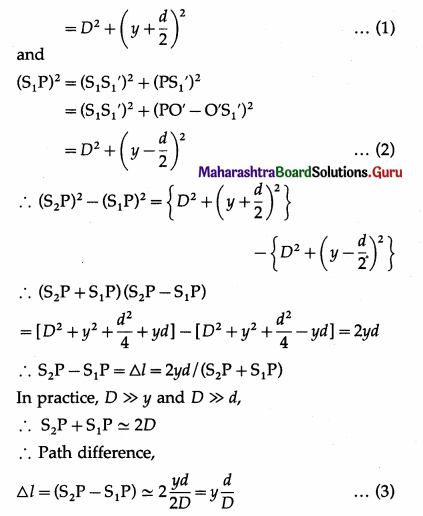Expression for the fringe width (or band width) : The distance between consecutive bright (or dark) fringes is called the fringe width (or band width) W. Point P will be bright (maximum intensity), if the path difference, ∆l = yn$$\frac{d}{D}$$ = nλ where n = 0,1, 2, 3…, Point P will be dark (minimum intensity equal to zero), if ym$$\frac{d}{D}$$ = (2m – 1)$$\frac{\lambda}{2}$$, where, m = 1, 2, 3…,
Thus, for bright fringes (or bands),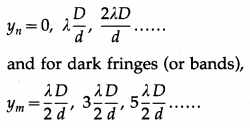These conditions show that the bright and dark fringes (or bands) occur alternately and are equally – spaced. For Point O’, the path difference (S2O’ – S1O’) = 0. Hence, point O’ will be bright. It corresponds to the centre of the central bright fringe (or band). On both sides of O’, the interference pattern consists of alternate dark and bright fringes (or band) parallel to the slit.

Let yn and yn + 1, be the distances of the nth and (n + 1)th bright fringes from the central bright fringe.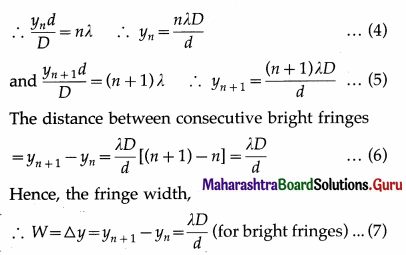Alternately, let ym and ym + 1 be the distances of the m th and (m + 1)th dark fringes respectively from the central bright fringe.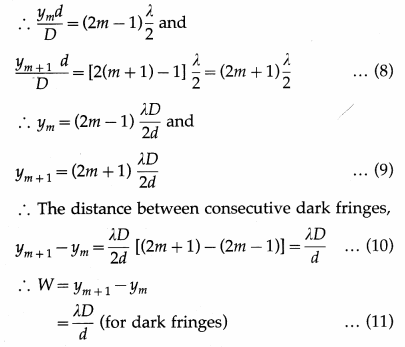Eqs. (7) and (11) show that the fringe width is the same for bright and dark fringes.

[Note : In the first approximation, the path difference is d sin θ.]Question 8.
What are the conditions for obtaining good interference pattern? Give reasons.
The conditions necessary for obtaining well defined and steady interference pattern :

1. The two sources of light should be coherent:
The two sources must maintain their phase relation during the time required for observation. If the phases and phase difference vary with time, the positions of maxima and minima will also change with time and consequently the interference pattern will change randomly and rapidly, and steady interference pattern would not be observed. For coherence, the two secondary sources must be derived from a single original source.
2. The light should be monochromatic :
Otherwise, interference will result in complex coloured bands (fringes) because the separation of successive bright bands (fringes) is different for different colours. It also may produce overlapping bands.
3. The two light sources should be of equal brightness, i.e., the waves must have the same amplitude.
The interfering light waves should have the same amplitude. Then, the points where the waves meet in opposite phase will be completely dark (zero intensity). This will increase the contrast of the interference pattern and make it more distinct.
4. The two light sources should be narrow :
If the source apertures are wide in comparison with the light wavelength, each source will be equivalent to multiple narrow sources and the superimposed pattern will consist of bright and less bright fringes. That is, the interference pattern will not be well defined.
5. The interfering light waves should be in the same state of polarization :
Otherwise, the points where the waves meet in opposite phase will not be completely dark and the interference pattern will not be distinct.
6. The two light sources should be closely spaced and the distance between the screen and the sources should be large : Both these conditions are desirable for appreciable fringe separation. The separation of successive bright or dark fringes is inversely proportional to the closeness of the slits and directly proportional to the screen distance.

Question 9.
What is meant by coherent sources? What are the two methods for obtaining coherent sources in the laboratory?
Coherent sources : Two sources of light are said to be coherent if the phase difference between the emitted waves remains constant.

It is not possible to observe interference pattern with light from any two different sources. This is because, no observable interference phenomenon occurs by superposing light from two different sources. This happens due to the fact that different sources emit waves of different frequencies. Even if the two sources emit light of the same frequency, the phase difference between the wave trains from them fluctuates randomly and rapidly, i.e., they are not coherent.
Consequently, the interference pattern will change randomly and rapidly, and steady interference pattern would not be observed.

In the laboratory, coherent sources can be obtained by using
(1) Lloyd’s mirror and
(2) Fresnel’s biprism.

(1) Lloyd’s mirror : A plane polished mirror is kept at some distance from the source of monochromatic light and light is made incident on the mirror at a grazing angle.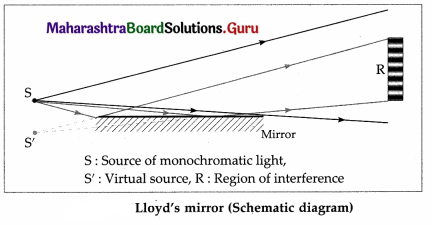Some light falls directly on the screen as shown by the black lines in above figure, while some light falls on the screen after reflection from the mirror as shown by red lines. The reflected light appears to come from a virtual source and thus two sources can be obtained. These two sources are coherent as they are derived from a single source. Superposition of the waves coming from these coherent sources, under appropriate conditions, gives rise to interference pattern consisting of alternate bright and dark bands on the screen as shown in the figure.

(2) Fresnel’s biprism : It is a single prism having an obtuse angle of about 178° and the other two angles of about 1° each. The biprism can be considered as made of two thin prisms of very small refracting angle of about 1°. The source, in the form of an illuminated narrow slit, is aligned parallel to the refracting edge of the biprism. Monochromatic light from the source is made to pass through that narrow slit and fall on the biprism.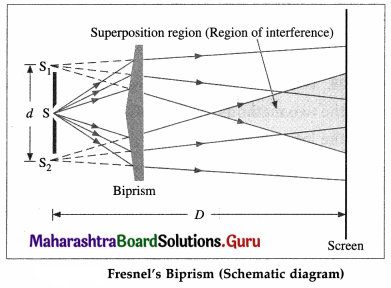Two virtual images S1 and S2 are formed by the two halves of the biprism. These are coherent sources which are obtained from a single secondary source S. The two waves coming from S1 and S2 interfere under appropriate conditions and form interference fringes, like those obtained in Young’s double-slit experiment, as shown in the figure in the shaded region. The formula for y is the same as in Young’s experiment.

Question 10.
What is diffraction of light? How does it differ from interference? What are Fraunhoffer and Fresnel diffractions?
1. Phenomenon of diffraction of light: When light passes by the edge of an obstacle or through a small opening or a narrow slit and falls on a screen, the principle of rectilinear propagation of light from geometrical optics predicts a sharp shadow. However, it is found that some of the light deviates from its rectilinear path and penetrates into the region of the geometrical shadow. This is a general characteristic of wave phenomena, which occurs whenever a portion of the wavefront is obstructed in some way. This bending of light waves at an edge into the region of geometrical shadow is called diffraction of light.

2. Differences between interference and diffraction :

1. The term interference is used to characterise the superposition of a few coherent waves (say, two). But when the superposition at a point involves a large number of waves coming from different parts of the same wavefront, the effect is referred to as diffraction.
2. Double-slit interference fringes are all of equal width. In single-slit diffraction pattern, only the non-central maxima are of equal width which is half of that of the central maximum.
3. In double-slit interference, the bright and dark fringes are equally spaced. In diffraction, only the non-central maxima lie approximately halfway between the minima.
4. In double-slit interference, bright fringes are of equal intensity. In diffraction, successive non-central maxima decrease rapidly in intensity.

[Note : Interference and diffraction both have their origin in the principle of superposition of waves. There is no physical difference between them. It is just a question of usage. When there are only a few sources, say two, the phenomenon is usually called interference. But, if there is a large number of sources the word diffraction is used.]

3. Diffraction can be classified into two types depending on the distances involved in the experimental setup :

(A) Fraunhofer diffraction : In this class of diffraction, both the source and the screen are at infinite distances from the aperture. This is achieved by placing the source at the focus of a convex lens and the screen at the focal plane of another convex lens.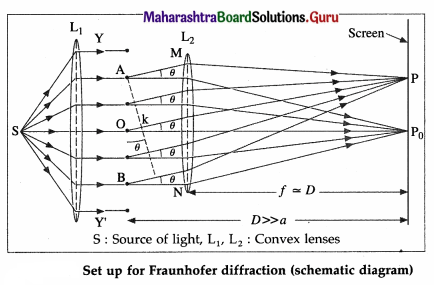(B) Fresnel diffraction : In this class of diffraction, either the source of light or the screen or both are at finite distances from the diffracting aperture. The incident wavefront is either cylindrical or spherical depending on the source. A lens is not needed to observe the diffraction pattern on the screen.

Question 11.
Derive the conditions for bright and dark fringes produced due to diffraction by a single slit.
When a parallel beam of monochromatic light of wavelength λ illuminates a single slit of finite width a, we observe on a screen some distance from the slit, a broad pattern of alternate dark and bright fringes. The pattern consists of a central bright fringe, with successive dark and bright fringes of diminishing intensity on both sides. This is called ‘ the diffraction pattern of a single slit.

Consider a single slit illuminated with a parallel beam of monochromatic light perpendicular to the plane of the slit. The diffraction pattern is obtained on a screen at a distance D (» a) from the slit and at the focal plane of the convex lens, Fig. 7.33.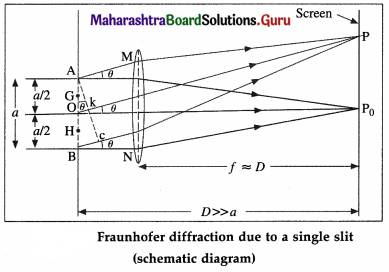We can imagine the single slit as being made up of a large number of Huygens’ sources evenly distributed over the width of the slit. Then the maxima and minima of the pattern arise from the interference of the various Huygens’ wavelets.

Now, imagine the single slit as made up of two adjacent slits, each of width a/2. Since, the incident plane wavefronts are parallel to the plane of the slit, all the Huygens sources at the slit will be in phase. They will therefore also in phase at the point P0 on the screen, where P0 is equidistant from all the Huygens sources. At P0, then, we get the central maximum.

For the first minimum of intensity on the screen, the path difference between the waves from the Huygens sources A and O (or O and B) is λ/2, which is the condition for destructive interference. Suppose, the nodal line OP for the first minimum subtends an angle θ at the slit; θ is very small. With P as the centre and PA as radius, strike an arc intersecting PB at C. Since, D » a, the arc AC can be considered a straight line at right angles to PB. Then, ∆ABC is a right-angled triangle similar to ∆OP0P.
This means that, ∠BAC = θ
∴ BC = a sinθ
∴ Difference in path length,
BC = PB – PA = (PB – PO) + (PO – PA)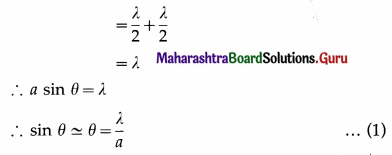(∵ θ is very small and in radian)
The other nodal lines of intensity minima can be understood in a similar way. In general, then, for the with minimum (m = ±1, ±2, ±3, …).
θm = $$\frac{m \lambda}{a}$$ (mth minimum) … (2)
as θm is very small and in radian.
Between the successive minima, the intensity rises to secondary maxima when the path difference is an odd-integral multiple of $$\frac{\lambda}{2}$$ :
a sin θm = (2m + 1)$$\frac{\lambda}{2}$$ = (m + $$\frac{1}{2}$$)λ
i.e., at angles given by,
θm $$\simeq$$ sin θm = (m + $$\frac{1}{2}$$)$$\frac{\lambda}{a}$$
(with secondary maximum) … (3)Question 12.
Describe what is Rayleigh’s criterion for resolution. Explain it for a telescope and a microscope.
Rayleigh’s criterion for minimum resolution : Two overlapping diffraction patterns due to two point sources are acceptably or just resolved if the centre of the central peak of one diffraction pattern is as far as the first minimum of the other pattern.

The ‘sharpness’ of the central maximum of a diffraction pattern is measured by the angular separation between the centre of the peak and the first minimum. It gives the limit of resolution.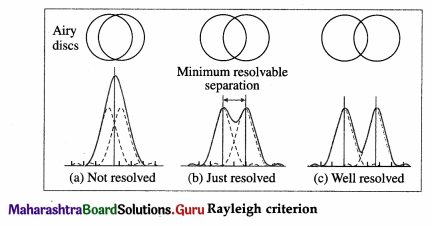Two overlapping diffraction patterns due to two point sources are not resolved if the angular separation between the central peaks is less than the limit of resolution. They are said to be just separate, or resolved, if the angular separation between the central peaks is equal to the limit of resolution. They are said to be well resolved if the angular separation between the central peaks is more than the limit of resolution.

Resolving power of an optical instrument:
The primary aim of using an optical instrument is to see fine details, whether observing a star system through a telescope or a living cell through a microscope. After passing through an optical system, light from two adjacent parts of the object should produce sharp, distinct (separate) images of those parts. The objective lens or mirror of a telescope or microscope acts like a circular aperture. The diffraction pattern of a circular aperture consists of a central bright spot (called the Airy disc and corresponds to the central maximum) and concentric dark and bright rings.

Light from two close objects or parts of an object after passing through the aperture of an optical system produces overlapping diffraction patterns that tend to obscure the image. If these diffraction patterns are so broad that their central maxima overlap substantially, it is difficult to decide if the intensity distribution is produced by two separate objects or by one.

The resolving power of an optical instrument, e.g., a telescope or microscope, is a measure of its ability to produce detectably separate images of objects that are close together.

Definition : The smallest linear or angular separation between two point objects which appear just resolved when viewed through an optical instrument is called the limit of resolution of the instrument and its reciprocal is called the resolving power of the instrument.

Rayleigh’s criterion for minimum resolution : Two overlapping diffraction patterns due to two point sources are acceptably or just resolved if the centre of the central peak of one diffraction pattern is as far as the first minimum of the other pattern.

The ‘sharpness’ of the central maximum of a diffraction pattern is measured by the angular separation between the centre of the peak and the first minimum. It gives the limit of resolution.Two overlapping diffraction patterns due to two point sources are not resolved if the angular separation between the central peaks is less than the limit of resolution. They are said to be just separate, or resolved, if the angular separation between the central peaks is equal to the limit of resolution. They are said to be well resolved if the angular separation between the central peaks is more than the limit of resolution.

The resolving power of a telescope is defined as the reciprocal of the angular limit of resolution between two closely-spaced distant objects so that they are just resolved when seen through the telescope.

Consider two stars seen through a telescope. The diameter (D) of the objective lens or mirror corresponds to the diffracting aperture. For a distant point source, the first diffraction minimum is at an angle θ away from the centre such that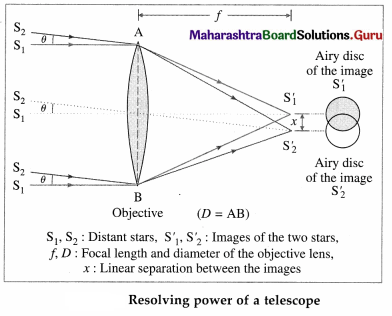Dsinθ = 1.22 λ
where λ is the wavelength of light. The angle θ is usually so small that we can substitute sin θ $$\approx$$ θ (θ in radian). Thus, the Airy disc for each star will be spread out over an angular half-width θ = 1.22 λ/D about its geometrical image point. The radius of the Airy disc at the focal plane of the objective lens is r = fθ = 1.22fλ/D, where / is the focal length of the objective.

When observing two closely-spaced stars, the Rayleigh criterion for just resolving the images as that of two point sources (instead of one) is met when the centre of one Airy disc falls on the first minimum of the other pattern. Thus, the angular limit (or angular separation) of resolution is
θ = $$\frac{1.22 \lambda}{D}$$ …. (1)
and the linear separation between the images at the focal plane of the objective lens is
y = fθ …(2)
∴ Resolving power of a telescope,
R = $$\frac{1}{\theta}$$ = $$\frac{D}{1.22 \lambda}$$ … (3)
It depends

1. directly on the diameter of the objective lens or mirror,
2. inversely on the wavelength of the radiation.

Question 13.
Whitelight consists of wavelengths from 400 nm to 700 nm. What will be the wavelength range seen when white light is passed through glass of refractive index 1.55? [Ans: 258.1 – 451.6 nm]
Let λ1 and λ2 be the wavelengths of light in water for 400 nm and 700 nm (wavelengths in vacuum) respectively. Let λa be the wavelength of light in vacuum.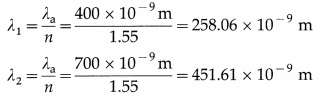The wavelength range seen when white light is passed through the glass would be 258.06 nm to 451.61 nm.

Question 14.
The optical path of a ray of light of a given wavelength travelling a distance of 3 cm in flint glass having refractive index 1.6 is same as that on travelling a distance x cm through a medium having refractive index 1.25. Determine the value of x.
[Ans: 3.84 cm]
Let dfg and dm be the distances by the ray of light in the flint glass and the medium respectively. Also, let nfg and nm be the refractive indices of the flint glass and the medium respectively.
Data : dfg = 3 cm, nfg = 1.6, nm = 1.25,
Optical path = nm × dm = nfg × dfg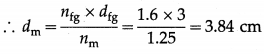Thus, x cm = 3.84 cm
∴ x = 3.84
This is the value of x.

Question 15.
A double-slit arrangement produces interference fringes for sodium light (λ = 589 nm) that are 0.20° apart. What
is the angular fringe separation if the entire arrangement is immersed in water (n = 1.33)? [Ans: 0.15°]
Data : θ1 = 0.20°, nw = 1.33
In the first approximation,
D sin θ1 = y1 and D sin θ2 = y2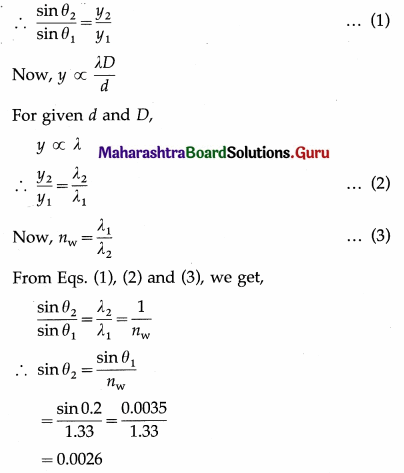∴ θ2 = sin-10.026
= 9′ = 0.15°
This is the required angular fringe separation.
OR
In the first approximation,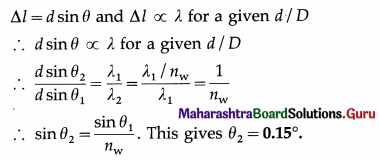Question 16.
In a double-slit arrangement the slits are separated by a distance equal to 100 times the wavelength of the light passing through the slits.
(a) What is the angular separation in radians between the central maximum and an adjacent maximum?
(b) What is the distance between these maxima on a screen 50.0 cm from the slits?
Data : d = 100λ, D = 50.0 cm
(a) The condition for maximum intensity in Young’s experiment is, d sin θ = nλ, n = 0, 1, 2 …,
The angle between the central maximum and its adjacent maximum can be determined by setting n equal to 1,
∴ d sin θ = λ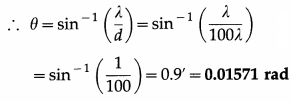(b) The distance between these maxima on the screen is D sin θ = D$$\left(\frac{\lambda}{d}\right)$$
= (50.0 cm)$$\left(\frac{\lambda}{100 \lambda}\right)$$
= 0.50 cmQuestion 17.
Unpolarized light with intensity I0 is incident on two polaroids. The axis of the first polaroid makes an angle of 50° with the vertical, and the axis of the second polaroid is horizontal. What is the intensity of the light after it has passed through the second polaroid? [Ans: I0/2 × (cos 40°)2]
According to Malus’ law, when the unpolarized light with intensity I0 is incident on the first polarizer, the polarizer polarizes this incident light. The intensity of light becomes I1 = I0/2.
Now, I2 = I1 cos2θ
∴ I2 = $$\left(\frac{I_{0}}{2}\right)$$ cos2θ
Also, the angle θ between the axes of the two polarizers is θ2 – θ1.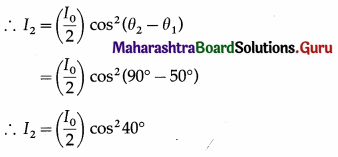The intensity of light after it has passed through the second polaroid = $$\left(\frac{\boldsymbol{I}_{0}}{\mathbf{2}}\right)$$cos240° = $$\frac{I_{0}}{2}$$(0.7660)2
= 0.2934 I0

Question 18.
In a biprism experiment, the fringes are observed in the focal plane of the eyepiece at a distance of 1.2 m from the slits. The distance between the central bright band and the 20th bright band is 0.4 cm. When a convex lens is placed between the biprism and the eyepiece, 90 cm from the eyepiece, the distance between the two virtual magnified images is found to be 0.9 cm. Determine the wavelength of light used. [Ans: 5000 Å]
Data : D = 1.2 m
The distance between the central bright band and the 20th bright band is 0.4 cm.
∴ y20 = 0.4 cm = 0.4 × 10-2 m
W = $$\frac{y_{20}}{20}$$ = $$\frac{0.4}{20}$$ × 10-2 m = 2 × 10-4 m,
d1 = 0.9 cm = 0.9 × 10-2m, v1 = 90 cm = 0.9 m
∴ u1 = D – v1 = 1.2m – 0.9m = 0.3 m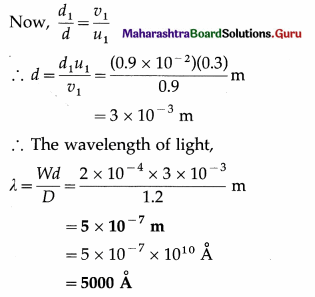Question 19.
In Fraunhoffer diffraction by a narrow slit, a screen is placed at a distance of 2 m from the lens to obtain the diffraction pattern. If the slit width is 0.2 mm and the first minimum is 5 mm on either side of the central maximum, find the wavelength of light. [Ans: 5000 Å]
Data : D = 2 m, y1d = 5 mm = 5 × 10-3 m, a = 0.2 mm = 0.2 × 10-3 m = 2 × 10-4 m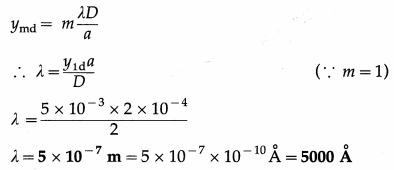This is the wavelength of light.

Question 20.
The intensity of the light coming from one of the slits in Young’s experiment is twice the intensity of the light coming from the other slit. What will be the approximate ratio of the intensities of the bright and dark fringes in the resulting interference pattern? [Ans: 34]
Data : I1 : I2 = 2 : 1
If E10 and E20 are the amplitudes of the interfering waves, the ratio of the maximum intensity to the minimum intensity in the fringe system is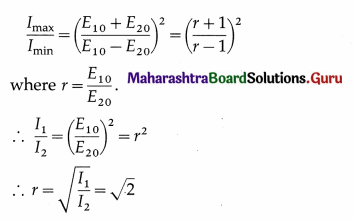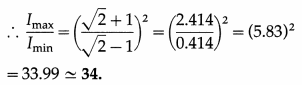The ratio of the intensities of the bright and dark fringes in the resulting interference pattern is 34 : 1.

Question 21.
A parallel beam of green light of wavelength 550 nm passes through a slit of width 0.4 mm. The intensity pattern of the transmitted light is seen on a screen which is 40 cm away. What is the distance between the two first order minima? [Ans: 1.1 mm]
Data : λ = 550 nm = 546 × 10-9 m, a = 0.4 mm = 4 × 10-4 m, D = 40 cm = 40 × 10-2 m
ymd = m$$\frac{\lambda D}{a}$$
∴ y1d = 1$$\frac{\lambda D}{a}$$ and
2y1d = $$\frac{2 \lambda D}{a}$$
= $$\frac{2 \times 550 \times 10^{-9} \times 40 \times 10^{-2}}{4 \times 10^{-4}}$$
= 2 × 550 × 10-6 = 1092 × 10-6
= 1.100 × 10-3m = 1.100 mm
This is the distance between the two first order minima.

Question 22.
What must be the ratio of the slit width to the wavelength for a single slit to have the first diffraction minimum at 45.0°? [Ans: 1.274]
Data : θ = 45°, m = 1
a sin θ = mλ for (m = 1, 2, 3… minima)
Here, m = 1 (First minimum)
∴ a sin 45° = (1) λ
∴ $$\frac{a}{\lambda}$$ = $$\frac{1}{\sin 45^{\circ}}$$ = 1.414
This is the required ratio.Question 23.
Monochromatic electromagnetic radiation from a distant source passes through a slit. The diffraction pattern is observed on a screen 2.50 m from the slit. If the width of the central maximum is 6.00 mm, what is the slit width if the wavelength is
(a) 500 nm (visible light);
(c) 0.500 nm (X-rays)?
[Ans: 0.4167 mm, 41.67 mm, 4.167 × 10-4 mm]
Data:2W = 6mm ∴ W= 3 mm = 3 × 10-3 m, y = 2.5 m,
(a) λ1 = 500 nm = 5 × 10-7 m
(b) λ2 = 50 μm = 5 × 10-5 m
(c) λ3 = 0.500 nm = 5 × 10-10 m
Let a be the slit width.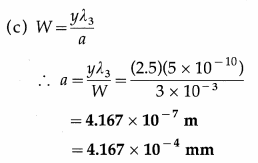Question 24.
A star is emitting light at the wavelength of 5000 Å. Determine the limit of resolution of a telescope having an objective of diameter of 200 inch. [Ans: 1.2 × 10-7 rad]
Data : λ = 5000 Å = 5 × 10-7 m,
D = 200 × 2.54 cm = 5.08 m
θ = $$\frac{1.22 \lambda}{D}$$
= $$\frac{1.22 \times 5 \times 10^{-7}}{5.08}$$
This is the required quantity.

Question 25.
The distance between two consecutive bright fringes in a biprism experiment using light of wavelength 6000 Å is 0.32 mm by how much will the distance change if light of wavelength 4800 Å is used? [Ans: 0.064 mm]
Data : λ1 = 6000 Å = 6 × 10-7m, λ2 = 4800 Å = 4.8 × 10-7m, W1 = 0.32 mm = 3.2 × 10-4m
Distance between consecutive bright fringes,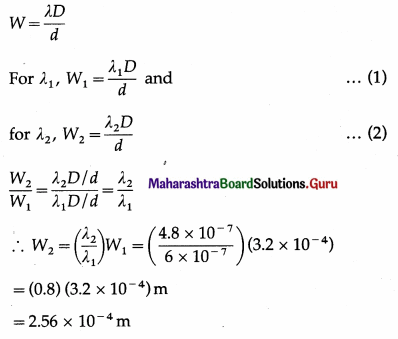∴ ∆W = W1 – W2
= 3.2 × 10-4m – 2.56 × 10-4m
= 0.64 × 10-4m
= 6.4 × 10-5
= 0.064 mm
This is the required change in distance.

12th Physics Digest Chapter 7 Wave Optics Intext Questions and Answers

Use your brain power (Textbook Page No. 167)

What will you observe if

Question 1.
you look at a source of unpolarized light through a polarizer ?
When a source of unpolarized light is viewed through a polarizer, the intensity of the light transmitted by the polarizer is reduced and hence the source appears less bright.Question 2.
you look at the source through two polarizers and rotate one of them around the path of light for one full rotation?
When a source of unpolarized light is viewed through two polarizers and the second polarizer is rotated gradually, the intensity of the light transmitted by the second polarizer goes on decreasing. When the axes of polarization of the two polarizers are at 90° to each other, light almost disappears depending on the quality of the polarizers. (Ideally the intensity of the transmitted light should be zero.) The light reappears, i.e., its intensity increases, when the second polarizer is rotated further, and the intensity of the light becomes maximum when the axes of polarization are parallel again.

Question 3.
instead of rotating only one of the polaroids, you rotate both polaroids simultaneously in the same direction?
If both the polaroids are rotated simultaneously in the same direction with the same angular velocity, then there would be no change in the intensity of the transmitted light observed.

Can you tell? (Textbook Page No. 168)

Question 1.
If you look at the sky in a particular direction through a polaroid and rotate the polaroid around that direction what will you see ?
As the scattered light is polarized, the sky appears bright and dim alternately.

Question 2.
Why does the sky appear to be blue while the clouds appear white ?
The blue colour of the sky is because of the scattering of light by air molecules and dust particles in the atmosphere. As the wavelength of blue light is less than that of red light, the blue light is preferentially scattered than the light corresponding to other colours in the visible region. Clouds are seen due to scattering of light from lower parts of the atmosphere. The clouds contain the dust particles and water droplets which are sometimes large enough to scatter light of all the wavelengths such that the combined effect makes the clouds appear white.

Remember this (Textbook Page No. 171)

Question 1.
For the interference pattern to be clearly visible on the screen, the distance (D) between the slits and the screen should be much larger than the distance (d) between the two slits (S1 and S2), i.e., D » d.
The condition for constructive interference at P is,
∆l = yn$$\frac{d}{D}$$ = nλ …. (1)
yn being the position (y-coordinate) of nth bright fringe (n = 0, ±1, ±2, …).
∴ yn = nλ$$\frac{D}{d}$$ ….. (2)
Similarly, the position of mth (m = +1, ±2,…) dark fringe (destructive interference) is given by,
∆l = ym$$\frac{d}{D}$$ = (2m – 1)λ giving
ym = (2m – 1)λ$$\frac{D}{d}$$ …(3)
The distance between any two consecutive bright or dark fringes, i.e., the fringe width
= W = ∆y = yn + 1 – yn = λ$$\frac{D}{d}$$ …(4)
Conditions given by Eqs. (1) to (4) and hence the location of the fringes are derived assuming that the two sources S1 and S2 are in phase. If there is a non-zero phase difference between them it should be added appropriately. This will shift the entire fringe pattern but will not change the fringe width.Do you know (Textbook Page No. 172 & 173)

Several phenomena that we come across in our day to day life are caused by interference and diffraction of light. These are the vigorous colours of soap bubbles as well as those seen in a thin oil film on the surface of water, the bright colours of butterflies and peacocks etc. Most of these colours are not due to pigments which absorb specific colours but are due to interference of light waves that are reflected by different layers.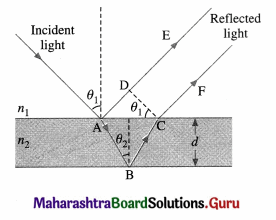Interference due to a thin film :
The brilliant colours of soap bubbles and thin films on the surface of water are due to the interference of light waves reflected from the upper and lower surfaces of the film. The two rays have a path difference which depends on the point on the film that is being viewed. This is shown in above figure.

The incident wave gets partially reflected from upper surface as shown by ray AE. The rest of the light gets refracted and travels along AB. At B it again gets partially reflected and travels along BC. At C it refracts into air and travels along CF. The parallel rays AE and CF have a phase difference due to their different path lengths in different media. As can be seen from the figure, the phase difference depends on the angle of incidence θ1 i.e., the angle of incidence at the top surface which is the angle of viewing, and also on the wavelength of the light as the refractive index of the material of the thin film depends on it. The two waves propagating along AE and CF interfere producing maxima and minima for different colours at different angles of viewing. One sees different colours when the film is viewed at different angles.

As the reflection is from the denser boundary, there is an additional phase difference of π radians (or an additional path difference λ). This should be taken into account for mathematical analysis.